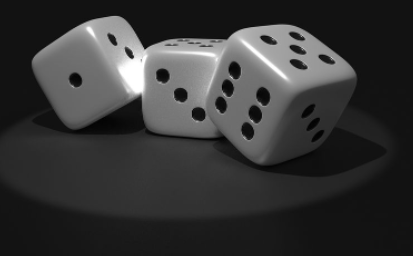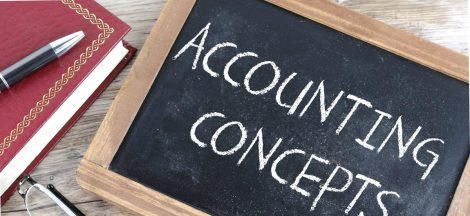Probability is a branch of mathematics concerning numerical descriptions of how likely an event is to occur, or how likely it is that a proposition is true. The probability of an event is a number between 0 and 1, where, roughly speaking, 0 indicates impossibility of the event and 1 indicates certainty. Probability is calculated by dividing the number of possible outcomes. After determining the probability event and its corresponding outcomes, divide the total number of events by the total number of possible outcomes. For instance, rolling a die once and landing on a three can be considered one event.

• Probability is a numerical measure of the likelihood that an event will occur
• Probability values are always assigned on a scale of 0 – 1
• A probability near 0 indicates an event is quite unlikely to occur
• A probability near 1 indicates an event is almost certain to occur

Probability is a statistical experiment – random event – not absolute

An experiment is any process is any process that generates well defined outcomes. The sample outcomes for an experiment are the set of all experimental outcomes. An experimental outcome is also called a sample point.

Events and their probability

An event is a collection of sample points. The probability of any event is equal to the sum of probabilities of the sample points in the event. One can identify all the sample points of an experiment and assign a probability to each.

The first step for calculating the probability of multiple events occurring at the same time is to determine each of the events you want to work with. For instance, one might calculate the probabilities of a rolling a six on two separate dice. Rolling each die separately represents one event. With this example, one will calculate the probabilities of these two events occurring at the same time.

Next, you can calculate the probability of rolling a six on one die, and the probability of rolling a six on the other die. The probability for each event results in a 1/6 chance that you roll a six with either die. Using these results, you can then find the total probability of these two events happening simultaneously.

Finally, one can multiply each probability together to get a total probability for all events that can occur. Using the dice example, one would calculate a total probability by multiplying the 1/6 chances you calculated in the above paragraph. Since each event has 1/6 chance of happening, one needs to multiply 1/6 x 1/6 to get a 1/36 chance of rolling a six on one die at the same time you roll a six with the other.

Why probability?

This is mainly for decision making. It could be used for various reasons ranging from decision analysis (with uncertainty), optimization (without uncertainty) usually used when seeking the best course of action especially in cases of maximizing profit. Another reason for probability is for sensitivity analysis. Probability can be used in a variety of situations, from creating sales forecasts to developing strategic marketing plans. It can be a highly useful tool for businesses who want to develop sound projections on things like sales, revenue and expected costs of operating a business.

Methods of probability

Classical method – number of independent outcomes (equally likely outcomes)

Relative frequency method – based on frequency, mode of occurrence with the weight.

That is:   X / sum of Xs

Subjective method – the subjective evaluation of probability of outcome. This method is based on judgement or estimate.

#MMBA3## A Beginner’s Guide to Interpreting Financial Statements

in## The Importance of the Cash Flow Statement for Business Owners and Managers #MMBA5

in